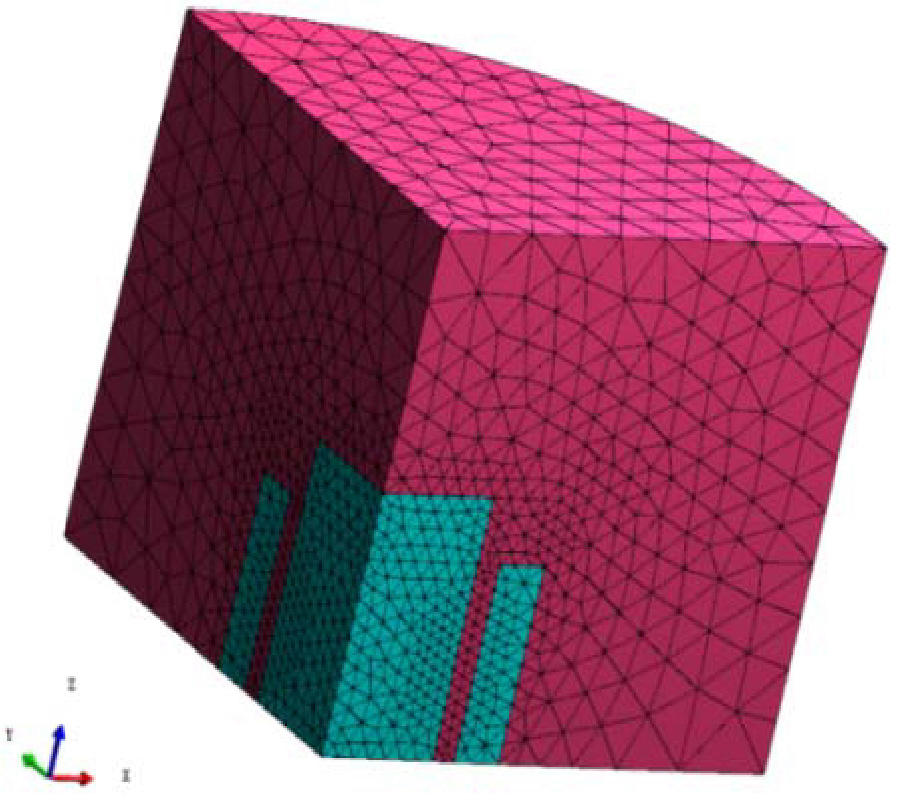# Vol. 64

Latest Volume
All Volumes
All Issues
2018-02-09

#### Compatible Finite Element Discretization of Generalized Lorenz Gauged Charge-Free a Formulation with Diagonal Lumping in Frequency and Time Domains

By Peng Jiang, Guozhong Zhao, Qun Zhang, and Zhenqun Guan
Progress In Electromagnetics Research M, Vol. 64, 167-179, 2018
doi:10.2528/PIERM17091803

## Abstract

The finite element implement of the generalized Lorenz gauged A formulation has been proposed for low-frequency modeling. However, the inverse of mass matrix of intermediate scalar in the finite element implement leads to additional computation cost and dense coefficient matrix. In this paper we propose to adopt a diagonal lumping mass matrix in the finite element discretization of the generalized Lorenz gauged double-curl operator in charge-free electromagnetic problems. Consequently, a sparser discrete system with improved condition number is thus obtained which is more favourable for low-frequency modeling in frequency-domain analysis. Furthermore, we apply the diagonal lumping formulation in time-domain analysis, showing that it can remedy spurious linear growth problem. Numerical examples are used to demonstrate the validity.

## Citation

Peng Jiang, Guozhong Zhao, Qun Zhang, and Zhenqun Guan, "Compatible Finite Element Discretization of Generalized Lorenz Gauged Charge-Free a Formulation with Diagonal Lumping in Frequency and Time Domains," Progress In Electromagnetics Research M, Vol. 64, 167-179, 2018.
doi:10.2528/PIERM17091803
http://test.jpier.org/PIERM/pier.php?paper=17091803

## References

1. Jin, J. M., The Finite Element Method in Electromagnetics, 3rd Ed., John Wiley & Sons, New York, 2015.

2. Lee, S. C., J. F. Lee, and R. Lee, "Hierarchical vector finite elements for analyzing waveguiding structures," IEEE Transactions on Microwave Theory and Techniques, Vol. 51, No. 8, 1897-1905, 2003.
doi:10.1109/TMTT.2003.815263

3. Lee, S. H. and J. M. Jin, "Application of the treecotree splitting for improving matrix conditioning in the full-wave finite-element analysis of high-speed circuits," Microwave and Optical Technology Letters, Vol. 50, No. 6, 1476-1481, 2008.
doi:10.1002/mop.23403

4. Zhu, J. and D. Jiao, "A theoretically rigorous full-wave finite-element-based solution of Maxwell’s equations from DC to high frequencies," IEEE Transactions on Advanced Packaging, Vol. 33, No. 4, 1043-1050, 2010.

5. Zhu, J. and D. Jiao, "A rigorous solution to the low-frequency breakdown in full-wave finiteelement- based analysis of general problems involving inhomogeneous lossless/lossy dielectrics and nonideal conductors," IEEE Transactions on Microwave Theory and Techniques, Vol. 59, No. 12, 3294-3306, 2011.
doi:10.1109/TMTT.2011.2171707

6. Venkatarayalu, N. V., et al., "Suppressing linear time growth in edge element based finite element time domain solution using divergence free constraint equation," Antennas and Propagation Society International Symposium, 193-196, 2005.

7. Hwang, C. T. and R. B.Wu, "Treating late-time instability of hybrid finite-element/finite-difference time-domain method," IEEE Transactions on Antennas and Propagation, Vol. 47, No. 2, 227-232, 1999.
doi:10.1109/8.761061

8. Golias, N. A. and T. D. Tsiboukis, "Magnetostatics with edge elements: A numerical investigation in the choice of the tree," IEEE Transactions on Magnetics, Vol. 30, No. 5, 2877-2880, 1994.
doi:10.1109/20.312537

9. Kikuchi, F., "Mixed and penalty formulations for finite element analysis of an eigenvalue problem in electromagnetism," Computer Methods in Applied Mechanics and Engineering, Vol. 64, No. 1, 509-521, 1987.

10. Chen, Z., Q. Du, and J. Zou, "Finite element methods with matching and nonmatching meshes for Maxwell equations with discontinuous coefficients," SIAM Journal on Numerical Analysis, Vol. 37, No. 5, 1542-1570, 2000.
doi:10.1137/S0036142998349977

11. Benzi, M., G. H. Golub, and J. Liesen, "Numerical solution of saddle point problems," Actanumerica, Vol. 14, 1-137, 2005.

12. Bespalov, A. N., "Finite element method for the eigenmode problem of a RF cavity resonator," Russian Journal of Numerical Analysis and Mathematical Modelling, Vol. 3, No. 3, 163-178, 1988.
doi:10.1515/rnam.1988.3.3.163

13. Hiptmair, R., "Finite elements in computational electromagnetism," Acta Numerica, Vol. 11, 237-339, 2002.

14. Chew, W. C., "Vector potential electromagnetics with generalized gauge for inhomogeneous media: Formulation," Progress In Electromagnetics Research, Vol. 149, 69-84, 2014.
doi:10.2528/PIER14060904

15. Li, Y. L., et al., "Finite element implementation of the generalized-Lorenz gauged A-Φ formulation for low-frequency circuit modeling," IEEE Transactions on Antennas and Propagation, Vol. 64, No. 10, 4355-4364, 2016.
doi:10.1109/TAP.2016.2593748

16. Li, Y. L., S. Sun, Q. I. Dai, and W. C. Chew, "Vectorial solution to double curl equation with generalized coulomb gauge for magnetostatic problems," IEEE Transactions on Magnetics, Vol. 51, No. 8, 1-6, 2015.
doi:10.1109/TMAG.2015.2423267

17. Bossavit, A. and L. Kettunen, "Yee-like schemes on a tetrahedral mesh, with diagonal lumping," International Journal of Numerical Modelling Electronic Networks Devices and Fields, Vol. 12, 129-142, 1999.
doi:10.1002/(SICI)1099-1204(199901/04)12:1/2<129::AID-JNM327>3.0.CO;2-G

18. Magele, C., H. Stogner, and K. Preis, "Comparison of different finite element formulations for 3D magnetostatic problems," IEEE Transactions on Magnetics, Vol. 24, No. 1, 31-34, 1988.
doi:10.1109/20.43846